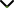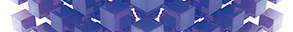# Applications of Fractional Calculus in the Nanofluid Flow Problems in a Porous Medium

Publishing date
01 May 2021
Status
Closed
25 Dec 2020

Guest Editors

1City University of Science and Information Technology, Peshawar, Pakistan

2Majmaah University, Al Majma'ah, Saudi Arabia

This issue is now closed for submissions.
More articles will be published in the near future.

# Applications of Fractional Calculus in the Nanofluid Flow Problems in a Porous Medium

This issue is now closed for submissions.
More articles will be published in the near future.

## DescriptionIn many physical and engineering processes, classical models of integer-order derivatives are unable to work requisitely in complex dynamics. In recent years, fractional calculus has been found to be convenient to describe such cases in chemistry, biology, mechanics, and signal and image processing. In fractional calculus, the derivatives of classical models are replaced by fractional order derivatives. There is a huge range of fractional models in the literature such as those used in fractional Brownian motion, fractional neutron point kinetic model, power law, Riesz potential, computational fractional derivative equations, fractional filters, fractional transforms, fractional wavelets, geophysics, fluid dynamics, and levy statistics. The most used among them are the Riemann-Liouville, Caputo, Caputo and Fabrizio, and Atangana-Baleanu fractional derivatives. Researchers have compared the solutions of the flow of a generalized fluid via Atangana-Baleanu and Caputo-Fabrizio fractional derivative approaches.

The work of fractional calculus is carried out for viscous fluids in a large number of articles, but few have considered fractional models for the flow of nanofluids. Nanofluids are of great importance in engineering and technology in the processes of heat transfer.

The aim of this Special Issue is to collate original research and review articles regarding the problem of analyzing nanofluids using different fractional derivatives.

Potential topics include but are not limited to the following:

• Modelling of fluid and nanofluid flow with fractional derivatives
• Exact solutions for nanofluid flow problems with fractional derivatives
• Numerical solutions
• Analytical solutions
• Experimental studies
• Comparison of different fractional operators
• Multiphase flows of generalized nanofluid flow problems
• Real world applications of generalized nanofluidsJournal metrics
Acceptance rate27%
Submission to final decision64 days
Acceptance to publication34 days
CiteScore1.800
Impact Factor1.305Author guidelinesEditorial boardDatabases and indexing

Article of the Year Award: Outstanding research contributions of 2020, as selected by our Chief Editors. Read the winning articles.